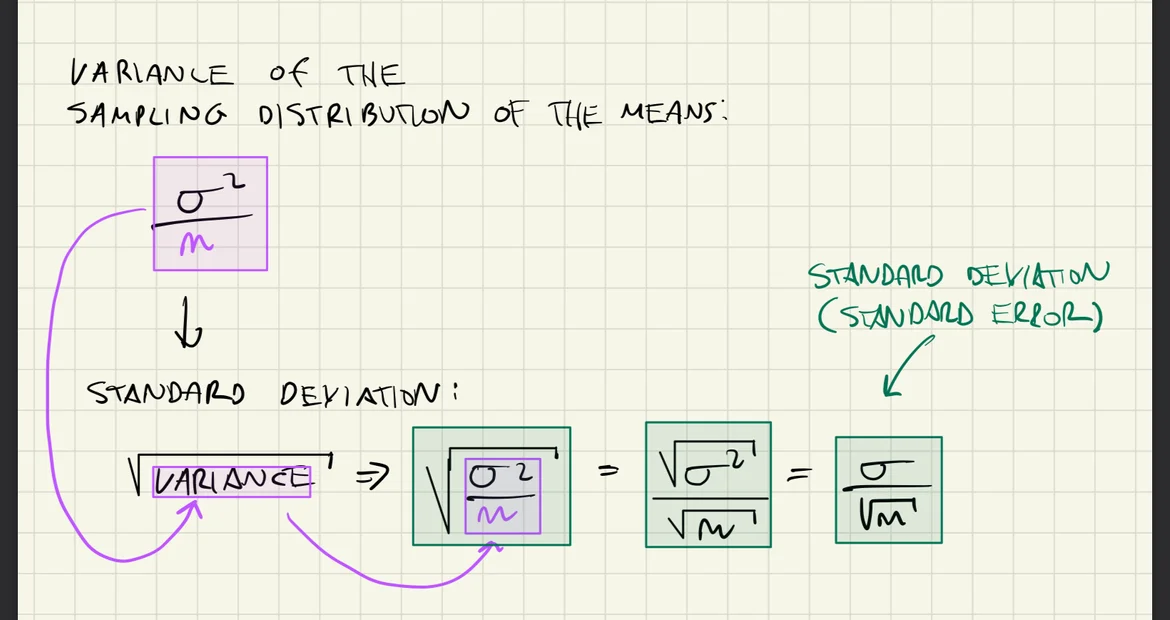13 Sept 2023

Posted on:

25 Oct 2022

0

# Resolved:why do you need to divide standard deviation of sampling means by sqrt of sample size?

why do you need to divide standard deviation of sampling means by sqrt of sample size? why cant it simply be the standard deviation of the sampling means distribution (this can also show the spread of means).
You say it is std. dev. of sampling means distribution, then just std. dev should be enough? but again why do you need to divide the sqrt of sample size?

Posted on:

25 Oct 2022

1

In theory, variance of sampling means distribution is variance of original population divided by sample size. But here you say, std. error it should be sqrt. of variance of sampling means distribution or the Std. dev. of sampling means distribution.

In the figure as per CLT, this is the variance of original population divided by sample size. This should be equal to std.dev of sampling means distribution. But then again to find the standard error, you  take the std. dev of sampling means (not of the  popln. and divide by sqrt of sample size)Posted on:

13 Jan 2023

0

"But then again to find the standard error, you  take the std. dev of sampling means (not of the  popln. and divide by sqrt of sample size)". Thwn you should take the population std dev.?

Posted on:

11 Sept 2023

0

In very simple words, the dataset formed by the means of several samples of size `n` you took from the population (also known as The Sampling Distribution of the means) has a variance of sigma^2/n (note that is not just sigma^2 but rather sigma^2 over `n`).

The standard deviation, which in this context is also the standard error is the square root of the variance. See pic below:Posted on:

13 Sept 2023

0

@Nishit Nishikant Did you find the answer to this? My head is spinning and going in circles trying to understand this. I believe that the stand deviation by itself for the sampling distribution should be the standard error, not devided by square root of n.